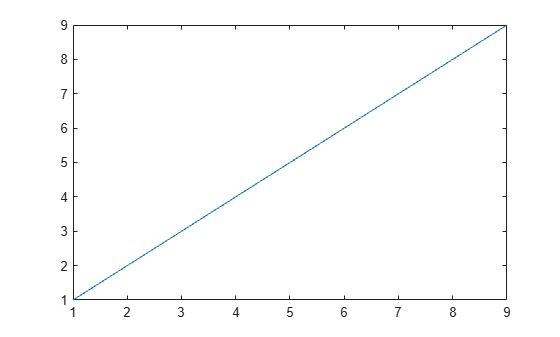Main Content

# Missing Data in MATLAB

Working with missing data is a common task in data preprocessing. Although sometimes missing values signify a meaningful event in the data, they often represent unreliable or unusable data points. In either case, MATLAB® has many options for handling missing data.

### Create and Organize Missing Data

The form that missing values take in MATLAB depends on the data type. For example, numeric data types such as `double` use `NaN` (not a number) to represent missing values.

`x = [NaN 1 2 3 4];`

You can also use the `missing` value to represent missing numeric data or data of other types, such as `datetime`, `string`, and `categorical`. MATLAB automatically converts the `missing` value to the data's native type.

`xDouble = [missing 1 2 3 4]`
```xDouble = 1×5 NaN 1 2 3 4 ```
`xDatetime = [missing datetime(2014,1:4,1)]`
```xDatetime = 1x5 datetime NaT 01-Jan-2014 01-Feb-2014 01-Mar-2014 01-Apr-2014 ```
`xString = [missing "a" "b" "c" "d"]`
```xString = 1x5 string <missing> "a" "b" "c" "d" ```
`xCategorical = [missing categorical({'cat1' 'cat2' 'cat3' 'cat4'})]`
```xCategorical = 1x5 categorical <undefined> cat1 cat2 cat3 cat4 ```

A data set might contain values that you want to treat as missing data, but are not standard MATLAB missing values in MATLAB such as `NaN`. You can use the `standardizeMissing` function to convert those values to the standard missing value for that data type. For example, treat 4 as a missing `double` value in addition to `NaN`.

`xStandard = standardizeMissing(xDouble,[4 NaN])`
```xStandard = 1×5 NaN 1 2 3 NaN ```

Suppose you want to keep missing values as part of your data set but segregate them from the rest of the data. Several MATLAB functions enable you to control the placement of missing values before further processing. For example, use the `'MissingPlacement'` option with the `sort` function to move `NaN`s to the end of the data.

`xSort = sort(xStandard,'MissingPlacement','last')`
```xSort = 1×5 1 2 3 NaN NaN ```

### Find, Replace, and Ignore Missing Data

Even if you do not explicitly create missing values in MATLAB, they can appear when importing existing data or computing with the data. If you are not aware of missing values in your data, subsequent computation or analysis can be misleading.

For example, if you unknowingly plot a vector containing a `NaN` value, the `NaN` does not appear because the `plot` function ignores it and plots the remaining points normally.

```nanData = [1:9 NaN]; plot(1:10,nanData)```However, if you compute the average of the data, the result is `NaN`. In this case, it is more helpful to know in advance that the data contains a `NaN`, and then choose to ignore or remove it before computing the average.

`meanData = mean(nanData)`
```meanData = NaN ```

One way to find `NaN`s in data is by using the `isnan` function, which returns a logical array indicating the location of any `NaN` value.

`TF = isnan(nanData)`
```TF = 1x10 logical array 0 0 0 0 0 0 0 0 0 1 ```

Similarly, the `ismissing` function returns the location of missing values in data for multiple data types.

`TFdouble = ismissing(xDouble)`
```TFdouble = 1x5 logical array 1 0 0 0 0 ```
`TFdatetime = ismissing(xDatetime)`
```TFdatetime = 1x5 logical array 1 0 0 0 0 ```

Suppose you are working with a table or timetable made up of variables with multiple data types. You can find all of the missing values with one call to `ismissing`, regardless of their type.

`xTable = table(xDouble',xDatetime',xString',xCategorical')`
```xTable=5×4 table Var1 Var2 Var3 Var4 ____ ___________ _________ ___________ NaN NaT <missing> <undefined> 1 01-Jan-2014 "a" cat1 2 01-Feb-2014 "b" cat2 3 01-Mar-2014 "c" cat3 4 01-Apr-2014 "d" cat4 ```
`TF = ismissing(xTable)`
```TF = 5x4 logical array 1 1 1 1 0 0 0 0 0 0 0 0 0 0 0 0 0 0 0 0 ```

Missing values can represent unusable data for processing or analysis. Use `fillmissing` to replace missing values with another value, or use `rmmissing` to remove missing values altogether.

`xFill = fillmissing(xStandard,'constant',0)`
```xFill = 1×5 0 1 2 3 0 ```
`xRemove = rmmissing(xStandard)`
```xRemove = 1×3 1 2 3 ```

Many MATLAB functions enable you to ignore missing values, without having to explicitly locate, fill, or remove them first. For example, if you compute the sum of a vector containing `NaN` values, the result is `NaN`. However, you can directly ignore `NaN`s in the sum by using the `'omitnan'` option with the `sum` function.

`sumNan = sum(xDouble)`
```sumNan = NaN ```
`sumOmitnan = sum(xDouble,'omitnan')`
```sumOmitnan = 10 ```

Download ebook